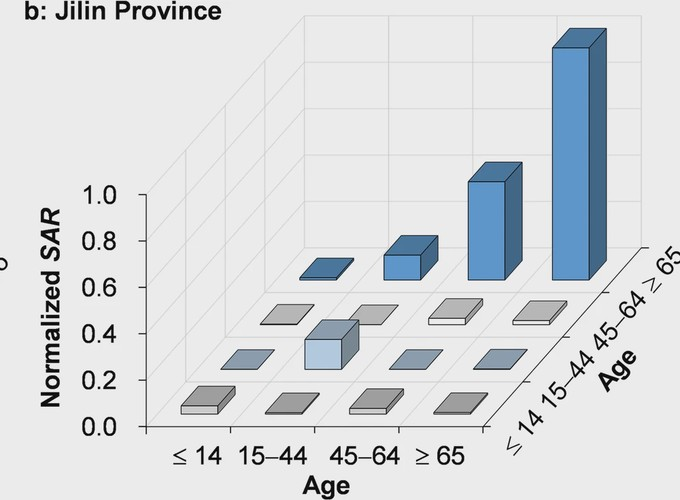Source

# A five-compartment model of age-specific transmissibility of SARS-CoV-2

### 摘要

Background The novel coronavirus, severe acute respiratory syndrome coronavirus 2 (SARS-CoV-2, also called 2019-nCoV) causes different morbidity risks to individuals in different age groups. This study attempts to quantify the age-specific transmissibility using a mathematical model. Methods An epidemiological model with five compartments (susceptible–exposed–symptomatic–asymptomatic–recovered/removed [SEIAR]) was developed based on observed transmission features. Coronavirus disease 2019 (COVID-19) cases were divided into four age groups: group 1, those ≤ 14 years old; group 2, those 15 to 44 years old; group 3, those 45 to 64 years old; and group 4, those ≥ 65 years old. The model was initially based on cases (including imported cases and secondary cases) collected in Hunan Province from January 5 to February 19, 2020. Another dataset, from Jilin Province, was used to test the model. Results The age-specific SEIAR model fitted the data well in each age group (P < 0.001). In Hunan Province, the highest transmissibility was from age group 4 to 3 (median: β43 = 7.71 × 10− 9; SAR43 = 3.86 × 10− 8), followed by group 3 to 4 (median: β34 = 3.07 × 10− 9; SAR34 = 1.53 × 10− 8), group 2 to 2 (median: β22 = 1.24 × 10− 9; SAR22 = 6.21 × 10− 9), and group 3 to 1 (median: β31 = 4.10 × 10− 10; SAR31 = 2.08 × 10− 9). The lowest transmissibility was from age group 3 to 3 (median: β33 = 1.64 × 10− 19; SAR33 = 8.19 × 10− 19), followed by group 4 to 4 (median: β44 = 3.66 × 10− 17; SAR44 = 1.83 × 10− 16), group 3 to 2 (median: β32 = 1.21 × 10− 16; SAR32 = 6.06 × 10− 16), and group 1 to 4 (median: β14 = 7.20 × 10− 14; SAR14 = 3.60 × 10− 13). In Jilin Province, the highest transmissibility occurred from age group 4 to 4 (median: β43 = 4.27 × 10− 8; SAR43 = 2.13 × 10− 7), followed by group 3 to 4 (median: β34 = 1.81 × 10− 8; SAR34 = 9.03 × 10− 8). Conclusions SARS-CoV-2 exhibits high transmissibility between middle-aged (45 to 64 years old) and elderly (≥ 65 years old) people. Children (≤ 14 years old) have very low susceptibility to COVID-19. This study will improve our understanding of the transmission feature of SARS-CoV-2 in different age groups and suggest the most prevention measures should be applied to middle-aged and elderly people.

Infectious Diseases of Poverty